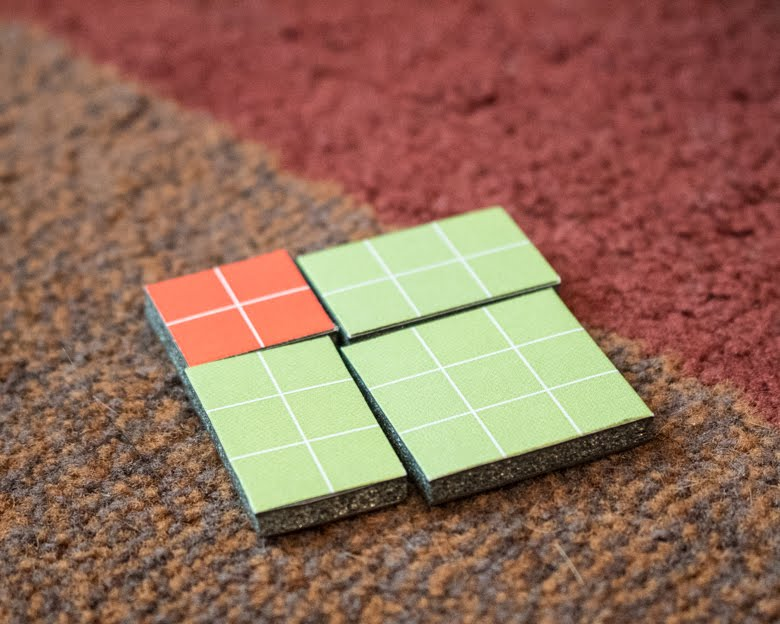## Saturday, May 13, 2017

### Homeschool Math: Use the Decanomial Square to Explore Binomial Squares

If you took algebra in middle school, you probably remember that squaring a binomial makes no sense.

Let's say you've got to square the expression (a+b). You square the first term, take twice the product of the two terms, and square the second term:

a squared + 2ab + b squared.

And you're all, "Umm... okay?" And your teacher is all, "Just memorize it. It'll be on the test."

And you do. And you earn an A on the test. And then you forget what you've just memorized, on account of it makes no sense and you have nowhere to put that information, nor anything to use it on other than more algebra.

Well, there's a deep, dark secret at the heart of Algebra 1, and that deep, dark secret is that the binomial square?

It is REAL:Will hasn't been asked to square binomials yet, and Syd certainly hasn't, either, so this is something that we're exploring well in advance, so that when each child is then asked to square her first binomial in her math curriculum, I can say, "Oh, that! Remember that we do that with the decanomial square. You remember what that looks like." Takes the fear out of math before the kid even knows there's something there to fear.

To start, I asked the kids to build the decanomial square and then to place the labels that let us use algebraic notation to name each piece of the square. Then, I asked each of them to pick two random squares from the decanomial square, and to place them kitty-corner to each other (kitty-corner, you probably know, is the official, scientific term). Then I asked them to figure out how to complete the puzzle to make a square:I did have to redirect Syd, as her first instinct was to piece together the sides with many small pieces. I told her that the perfect solution would be just two pieces, one for each side.

And you can see as well as I can what both kids discovered, that it's two identical pieces that complete this square:

If they don't quite notice that these two pieces have the same lengths as square a and square b, they do notice that as soon as I ask them to write down the expression that represents what they have:

You can see in the image above (you can also see that I need to recondition our very old dry erase board) that Will has written the expression correctly: c squared + cb + bc + b squared.

She's written "cb" and "bc" because that's how they're labeled on the square, one in row c and column b, and one in row b and column c. But she can see that they're perfectly identical, so that and her knowledge of the commutative property of multiplication (a*b=b*a) allows her to simplify cb+bc into 2cb.

The simplified expression, then is this:You can also look at the square that you've made and pick another way to write the expression, one that's even simpler: each side is made of length a + length e, so to represent the square as a whole, you can simply write (a+e) squared.

(a+e) squared = a squared + 2ae + e squared

Congratulations! You've just squared your first binomial!

See, that wasn't so hard, was it?